Next: Plasma Fluid Theory Up: Charged Particle Motion Previous: Third Adiabatic Invariant

# Motion in Oscillating Fields

We have seen that charged particles can be confined by a static magnetic field. A somewhat more surprising fact is that charged particles can also be confined by a rapidly oscillating, inhomogeneous electromagnetic wave-field. In order to demonstrate this, we again make use of our averaging technique. To lowest order, a particle executes simple harmonic motion in response to an oscillating wave-field. However, to higher order, any weak inhomogeneity in the field causes the restoring force at one turning point to exceed that at the other. On average, this yields a net force which acts on the centre of oscillation of the particle.

Consider a spatially inhomogeneous electromagnetic wave-field oscillating at frequency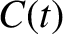: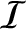(151)

The equation of motion of a charged particle placed in this field is written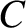(152)

where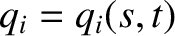(153)

In order for our averaging technique to be applicable, the electric field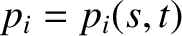experienced by the particle must remain approximately constant during an oscillation. Thus,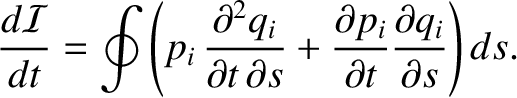(154)

When this inequality is satisfied, Eq. (153) implies that the magnetic force experienced by the particle is smaller than the electric force by one order in the expansion parameter. In fact, Eq. (154) is equivalent to the requirement,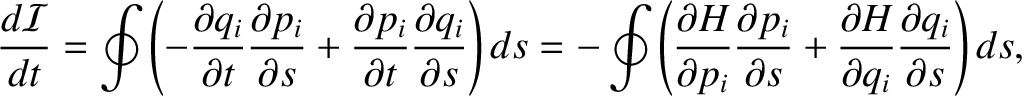, that the particle be unmagnetized.

We now apply the averaging technique. We make the substitution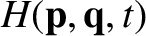in the oscillatory terms, and seek a change of variables,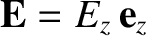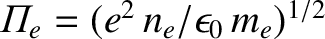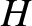(155)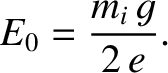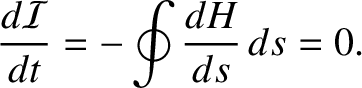(156)

such that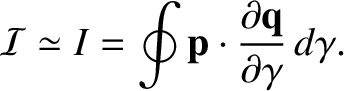and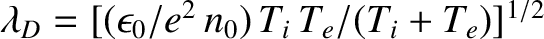are periodic functions ofwith vanishing mean. Averaging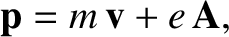again yields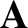to all orders. To lowest order, the momentum evolution equation reduces to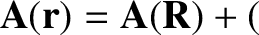(157)

The solution, taking into account the constraints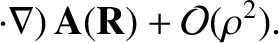, is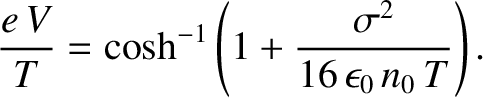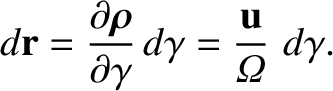(158)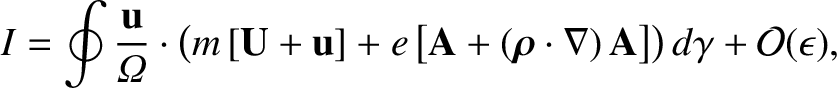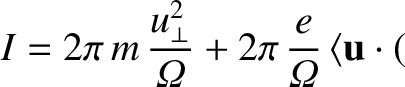(159)

Here,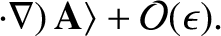represents an oscillation average.

Clearly, there is no motion of the centre of oscillation to lowest order. To first order, the oscillation average of Eq. (152) yields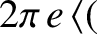(160)

which reduces to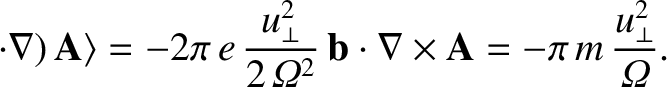(161)

The oscillation averages of the trigonometric functions are both equal to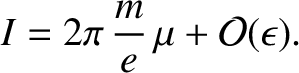. Furthermore, we have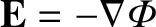. Thus, the equation of motion for the centre of oscillation reduces to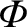(162)

where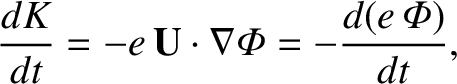(163)

It is clear that the oscillation centre experiences a force, called the ponderomotive force, which is proportional to the gradient in the amplitude of the wave-field. The ponderomotive force is independent of the sign of the charge, so both electrons and ions can be confined in the same potential well.

The total energy of the oscillation centre,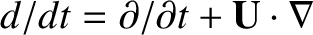(164)

is conserved by the equation of motion (161). Note that the ponderomotive potential energy is equal to the average kinetic energy of the oscillatory motion: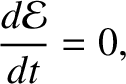(165)

Thus, the force on the centre of oscillation originates in a transfer of energy from the oscillatory motion to the average motion.

Most of the important applications of the ponderomotive force occur in laser plasma physics. For instance, a laser beam can propagate in a plasma provided that its frequency exceeds the plasma frequency. If the beam is sufficiently intense then plasma particles are repulsed from the centre of the beam by the ponderomotive force. The resulting variation in the plasma density gives rise to a cylindrical well in the index of refraction which acts as a wave-guide for the laser beam.Next: Plasma Fluid Theory Up: Charged Particle Motion Previous: Third Adiabatic Invariant
Richard Fitzpatrick 2011-03-31